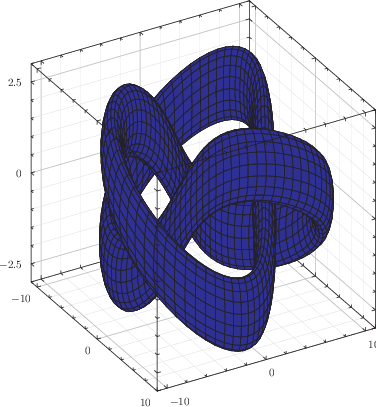## 8.6.1 Two-dimensional parametric surfaces

Pyxplot can also plot datasets which can be parameterised in terms of two free parametersand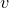. This is most often useful in conjunction with the surface plot style, allowing any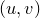-surface to be plotted (see Section 8.14.1 for details of the surface plot style). However, it also works in conjunction with any other plot style, allowing, for example,-grids of points to be constructed.

As in the case of parametric lines above, the range of values that each free parameter should take must be specified. This can be done using the set urange and set vrange commands. These commands also act to switch Pyxplot between one- and two-dimensional parametric function evaluation; whilst the set trange command indicates that the next parametric function should be evaluated along a single raster of values of, the set urange and set vrange commands indicate that a grid ofvalues should be used. By default, the range of values used forandis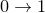.

The number of samples to be taken can be specified using a command of the form

set sample grid 20x50


which specifies that 20 different values ofand 50 different values ofshould be used, yielding a total of 1000 datapoints. The following example uses the lines plot style to generate a sequence of cross-sections through a two-dimensional Gaussian surface:

set num err quiet
set nokey
set size 7 square
set sample grid 20x20
set urange [-1:1] ; set vrange [-1:1]
set xrange [-1.1:1.1]
f(u,v) = 0.4*exp(-(u**2+v**2)/0.2)
plot parametric u:v+f(u,v) with l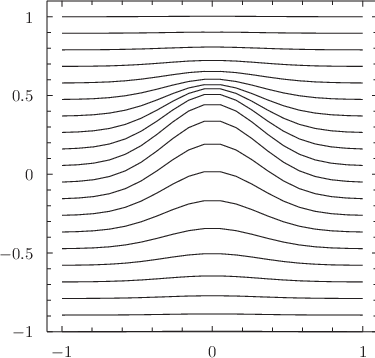The ranges of values to use forandmay alternatively be specified on a dataset-by-dataset basis within the plot command, as in the example

plot parametric [0:1][0:1] u:v , \
parametric [0:1] sin(t):cos(t)


Example: A three-dimensional view of a torus

In this example we plot a torus, which can be parametrised in terms of two free parametersandas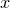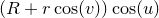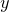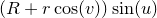whereandboth run in the range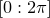,is the distance of the tube’s center from the center of the torus, and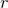is the radius of the tube.
R = 3
r = 0.5
f(u,v) = (R+r*cos(v))*cos(u)
g(u,v) = (R+r*cos(v))*sin(u)
h(u,v) = r*sin(v)

set urange [0:2*pi]
set vrange [0:2*pi]
set zrange [-2.5:2.5]

set nokey
set size 8 square
set grid
set sample grid 50x20
plot 3d parametric f(u,v):g(u,v):h(u,v) with surf fillcol blue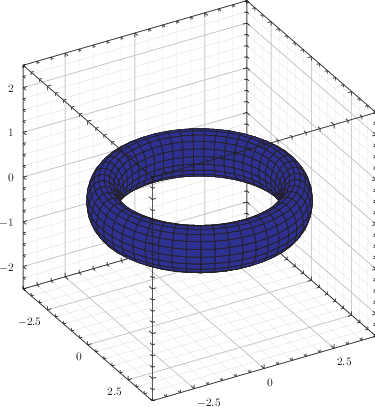Example: A three-dimensional view of a trefoil knot

In this example we plot a trefoil knot, which is the simplest non-trivial knot in topology. Simply put, this means that it is not possible to untie the knot without cutting it. The knot follows the line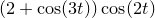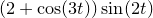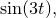but in this example we construct a tube around this line using the following parameterisation: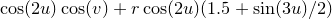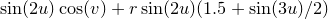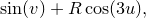whereandrun in the rangesandrespectively, andanddetermine the size and thickness of the knot as in an analogous fashion to the previous example.
r = 5
R = 2
f(u,v) = cos(2*u)*cos(v) + r*cos(2*u)*(1.5+sin(3*u)/2)
g(u,v) = sin(2*u)*cos(v) + r*sin(2*u)*(1.5+sin(3*u)/2)
h(u,v) = sin(v)+R*cos(3*u)

set urange [0:2*pi]
set vrange [-pi:pi]

set nokey
set size 8 square
set grid
set sample grid 150x20
plot 3d parametric f(u,v):g(u,v):h(u,v) with surf fillcol blue